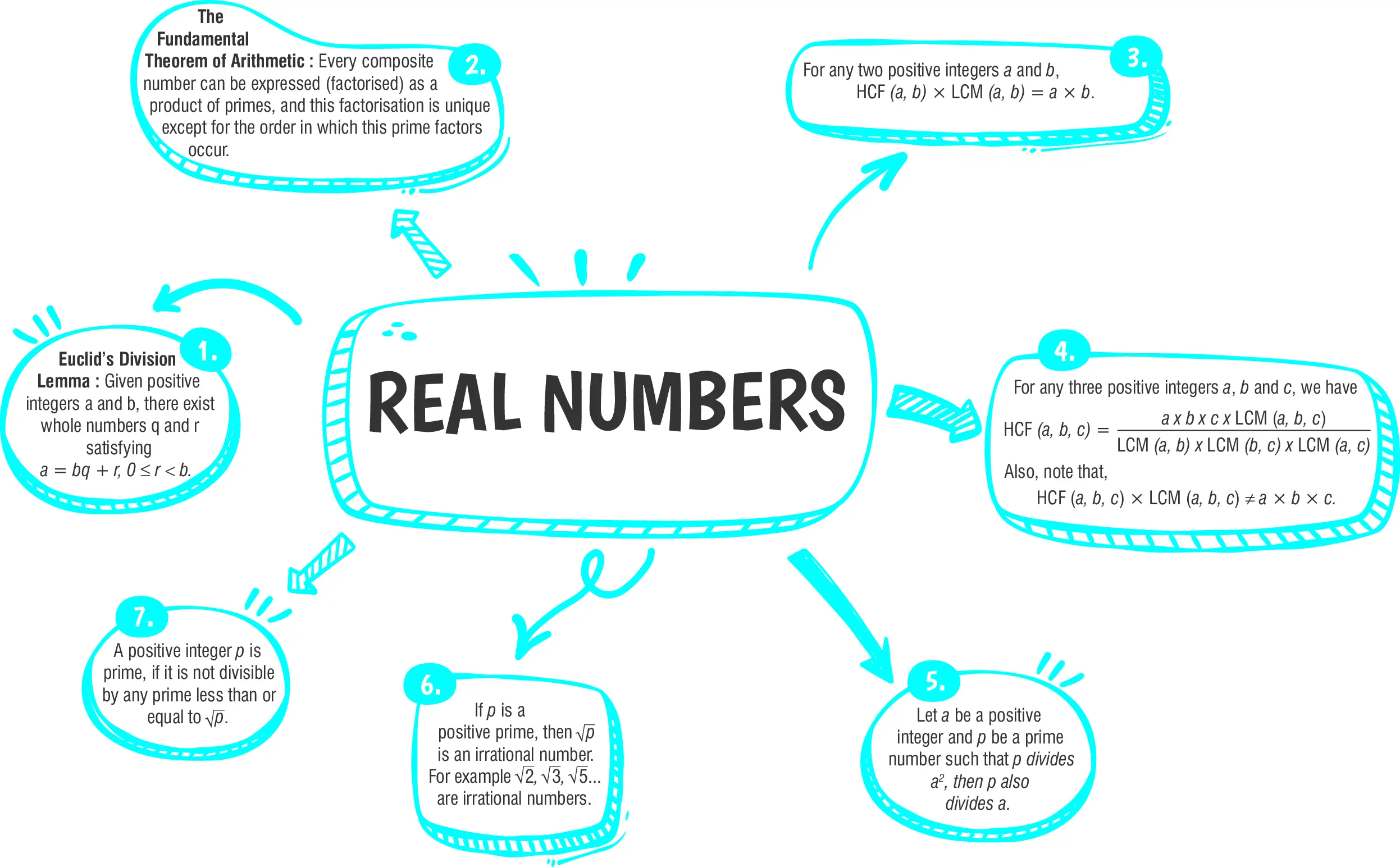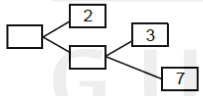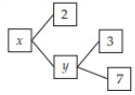Real Number

# Class 10 Maths Chapter 1 Real Numbers Important QuestionsHere are some important Class 10 Mathematics questions for Chapter 1, Real Numbers. These questions are designed to help students practice and score well in their CBSE Class 10 Mathematics Examination 2023-24. Practicing a variety of question types will help students clarify their doubts and prepare effectively for the exams. Solving these questions will not only build confidence but also enhance problem-solving skills.

## Introduction

In Chapter 1 of Class 10 Mathematics, Real Numbers, you will review operations with real numbers from previous grades and learn about two significant properties of positive integers: Euclid's Division Lemma and algorithm, as well as the fundamental theorem of arithmetic.

### What are Real Numbers?

Real numbers encompass positive integers, negative integers, irrational numbers, and fractions, with the exception of complex numbers. This means that almost every number falls under the category of real numbers. Examples of real numbers include -1, ½, 1.75, √2, and others.

• Real numbers consist of both rational and irrational numbers combined.
• Every real number can be represented on the number line.

Let's take a quick look at the chapter's summary.## Class 10 Real Numbers Important Questions and Answers

#### (a) 3(b) 4(c) 5(d) 2

Ans. (b)

Explanation:
Prime factorization is the method of representing a number as the multiplication of its prime factors. A number qualifies as prime if it possesses solely two factors , 1 and the number itself.Here's a breakdown of the sequential procedure for identifying the prime factors in the prime factorization of 196:
Prime factors of 196 = 22 ×  7
So, sum of exponents = 2+2=4

#### Q 2. Complete the missing entries in the following factor tree:#### (a) 42 and 21(b) 24 and 12(c) 7 and 3(d) 84 and 42

Ans. (a) 42 and 21

Explanation:
Using the factor tree, we have:From the above factor tree. It is clear that
y = 3 ×7 = 21
and                      x = 2 × y = 2 × 21
= 42.

#### Q 3.  The LCM of two numbers is 14 times their HCF. The sum of LCM and HCF is 750. If one number is 250, then find the other number.

Ans. 140

Explanation:
Let HCF be H.
Then                    LCM =14H
Now, sum of LCM and HCF is 750.                                          [Given]
$$∴ 14H + H = 750$$
$$⇒ 15H = 750$$
$$⇒ H =\Large\frac{750}{15}$$
$$⇒ H = 50$$
$$∴ LCM = 14H =14 × 50 = 700$$
We know that,
Product of LCM and HCF = Product of two numbers.
Then,                          700 × 50 = 250 × y
$$⇒ y = \large\frac{700 × 50}{250}$$
⇒                                y = 140
Hence, the other number is 140.

#### Q 4. On a morning walk, three men step off together and their steps measure 54 cm, 60 cm and 48 cm, respectively. What is the minimum distance each should walk so that each can cover the same distance in complete steps?

Ans. 21 m 60 cm

Explanation:
The minimum distance that each should walk is the LCM of 54, 60 and 48.
Now, 54 = 2 × 33
60 = 22×3×5
48 = 24 × 3
∴ LCM (54, 60, 48) = 24 × 33 × 5
= 2160
∴        Required distance  = 2160 cm
= 21 m 60 cm

#### Q 5. The LCM of two numbers is M times their HCF. The sum of LCM and HCF is 750. If one number is 250, then find the other number.

Ans. 140

Explanation:
Let HCF be ‘H’
Then             LCM =14H
sum of LCM and HCF is 750.
∴             14H + H  = 750
⇒                    15H = 750
$$\Rightarrow\text{H} =\large\frac{750}{15}$$
⇒                        H = 50
∴                    LCM = 14H
= 14×50
= 700
We know
Product of LCM and HCF = Product of two numbers.
Let other number be y
Then,           700 × 50 = 250 × y
$$⇒ \text{y} = \large\frac{700 × 50}{250}$$
⇒                                y = 140
Hence, the other number is 140.## CBSE Class 10 Maths Chapter wise Important Questions

Chapter No. Chapter Name
Chapter 1 Real Number
Chapter 2 Polynomials
Chapter 3 Pair of Linear Equations in Two Variables
Chapter 5 Arithmetic Progressions
Chapter 6 Triangles
Chapter 7 Coordinate Geometry
Chapter 8 Introduction to Trigonometry
Chapter 9 Some Applications of Trigonometry
Chapter 10 Circles
Chapter 11 Areas Related to Circle
Chapter 12 Surface Areas and Volumes
Chapter 13 Statistics
Chapter 14 Probability

#### Conclusion

If you are looking to further practice and enhance your understanding of the concepts discussed in the chapter, oswal.io provides a comprehensive set of questions for understanding the concept in a better way.

### Q1: What is the difference between rational and irrational numbers?

Ans: Rational numbers can be expressed as a fraction of two integers, while irrational numbers cannot. Rational numbers have terminating or repeating decimal representations, whereas irrational numbers have non-repeating, non-terminating decimal representations.

### Q2: Can real numbers be negative?

Ans: Yes, real numbers can be negative. They include negative rational numbers (e.g., -1/2, -3/4) and negative irrational numbers (e.g., -√2, -π).

### Q3: Are all integers real numbers?

Ans: Yes, all integers (positive, negative, and zero) are real numbers. In fact, integers are a subset of rational numbers because they can be expressed as fractions with a denominator of 1.

### Q4: What is the decimal representation of a real number?

Ans: The decimal representation of a real number is the representation of that number using decimal digits. It can be terminating (ends) or non-terminating (repeating or non-repeating).

### Q5: Are all square roots irrational?

Ans: No, not all square roots are irrational. The square root of perfect squares (like 4, 9, 16, etc.) is rational, while the square root of non-perfect squares (like 2, 3, 5, etc.) is irrational.

###### Copyright 2022 OSWAL PUBLISHERS Simplifying ExamsPhone:  (+91) 78959 87722
Mail: support@oswalpublishers.in